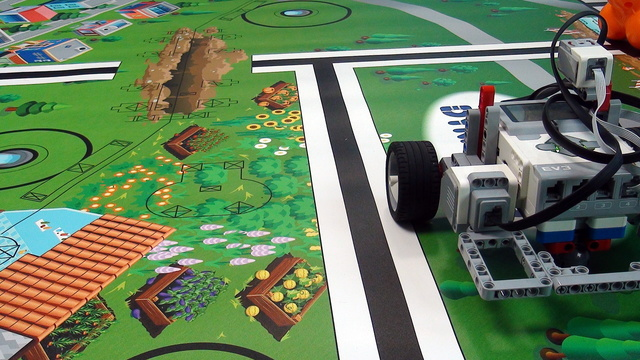# Implementing a "WaitForTick" logic in your EV3-G program ProPreview

Sometimes when we are working with sensors it is important that the time between two consecutive samples is the same. This will make each sample equally important and independent of how much time it took to take it. In this video tutorial, we would use the EV3-G timer block to make a "WaitForTick" program where the time between each sample of the EV3 Gyro takes exactly 0.02 seconds.

• #660
• 10 Jan 2018
• 3:01

### Program for returning to the Straight line after the Robot makes an error (Integral compensating)

This is an EV3-G project that contains two programs implementing an Integral compensation - integral part of the PID algorithm. The first program is for a Five Minute Bot and the second program is for Box Robot. The things that you should be careful when using the program for your robot are the direction of the motors in the steering block; whether the motors in the steering block are written as "B+C" or "C+B" and the coefficients in the two math blocks. The coefficients that we've chosen should work for most of the robots, but will probably not work for some of them. If they don't work, write to us, comment below in the comment section or drop us an email.

### English

Sometimes when we are working with sensors like the gyro sensor here it is important that the time between the different samples - different consecutive samples is the same. This will make each sample equally important and independent on how much time it took to take it. In this video tutorial we would use the EV3-G timer block to make a Wait for tick program where the time between each sample of the EV3 gyro takes exactly 0.02 seconds. This is actually quite simple to implement and what I'll do is to delete everything in our program like this and I'll just leave these two blocks. And these are the two blocks that we need. First, at the beginning of the loop we reset one of the timers and we have like 8 timers so we reset one of the timers and then at the end of the loop we have this wait block. And in this wait block we wait for timer 1 to become equal to or more than 0.1 seconds or as I said in the introduction we can make it to 0.02 seconds. And we can put some logic in between. We can put some logic for detecting the gyro sensor or the color sensor or some other sensors and some calculations and what will happen is that the timer will reset these calculations and these samples will be taken and at the end when the time is less than 0.02 seconds, we'll just wait. And this will make sure that each sample takes at least 0.02 seconds or at least 0.1 second. And this makes all the samples equally important. Because sometimes the gyro sensor or the color sensor might return the value after 0.01 second or after 0.02 seconds we don't know this and this very much is out of our control but what we want to have when we doing an experiment is to have each value equally important and each sample equally important. So, we say: We don't want to have a value that's taken just as a very quick sample. We want to have each sample taken after at least 0.01 second. So, these are a hundred milliseconds.

## Courses and lessons with this Tutorial

This Tutorial is used in the following courses and lessons• 24
• 102:09
• 3• 5
• 0
• 0
• 3d_rotation 1